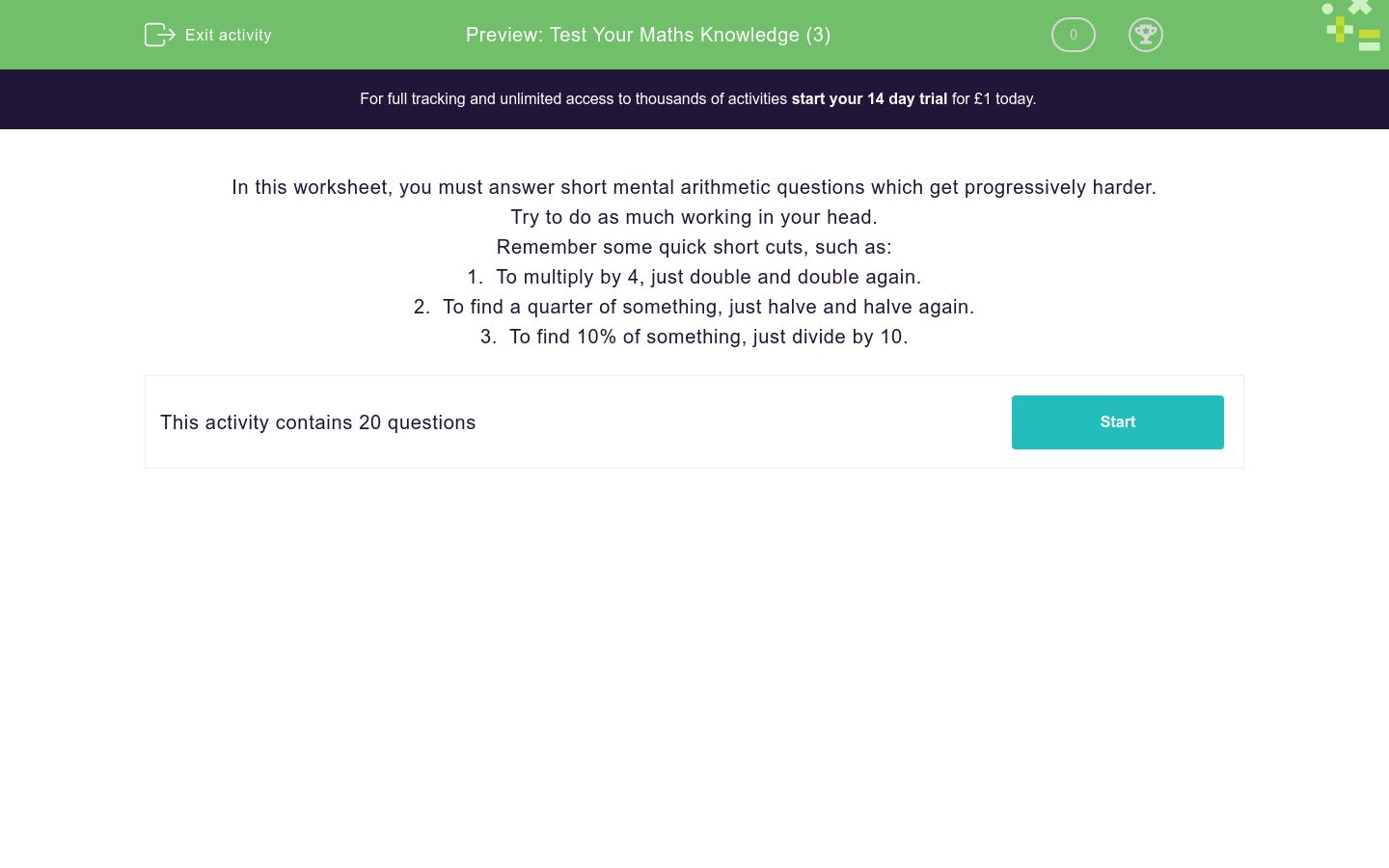# Test Your Maths Knowledge (3)

In this worksheet, students answer these mixed questions as quickly and accurately as possible. Most can be answered without writing anything down.Key stage:  KS 3

Curriculum topic:  Number

Curriculum subtopic:  Use Four Operations for All Numbers

Difficulty level:### QUESTION 1 of 10

In this worksheet, you must answer short mental arithmetic questions which get progressively harder.

Remember some quick short cuts, such as:

1.  To multiply by 4, just double and double again.

2.  To find a quarter of something, just halve and halve again.

3.  To find 10% of something, just divide by 10.

Subtract 38 from 300.

What is one-third of 57?

How many sides does a heptagon have?

A table has a height of 85 cm.

Write this height in metres.

What is the approximate mass of a drawing pin?

1g

10g

1kg

What is 0.8 written as a fraction in its lowest terms?

(write a fraction in the form a/b)

Twenty-four thousand, four hundred and forty-nine people attend a football match.

Write this number to the nearest hundred.

Work out two to the power of four divided by four squared.

If n equals 8, what is the value of 2n2?

Work out 12 + 3 x 6

The temperature at noon was 12 °C.  By midnight, it had fallen by 20 °C.  What was the temperature at midnight?

(just write the number)

The two equal angles of an isosceles triangle are each 52º.

What is the size of the third angle in degrees?

(just write the number)

A kilogram of potatoes costs 84p.

What is the cost of two hundred and fifty grams of potatoes?

Calculate the circumference of a circle of diameter 10cm.

What is the highest common factor of 32 and 80?

A fair die is numbered from 1 to 6.

What is the probability that it will show a square number when it is thrown?

How many prime numbers are there between 50 and 60 inclusive?

In a sale, 15% is taken off all prices.

What is the sale price of a shirt originally costing £40?

(just write the number)

A map is drawn to a scale of one to twenty-five thousand.  How many centimetres on the map represent one kilometre?

(Just write the number)

How many even two-digit square numbers are there?

• Question 1

Subtract 38 from 300.

262
• Question 2

What is one-third of 57?

19
• Question 3

How many sides does a heptagon have?

7
• Question 4

A table has a height of 85 cm.

Write this height in metres.

0.85 m
0.85m
• Question 5

What is the approximate mass of a drawing pin?

1g
• Question 6

What is 0.8 written as a fraction in its lowest terms?

(write a fraction in the form a/b)

4/5
• Question 7

Twenty-four thousand, four hundred and forty-nine people attend a football match.

Write this number to the nearest hundred.

24400
• Question 8

Work out two to the power of four divided by four squared.

1
EDDIE SAYS
16 ÷ 16
• Question 9

If n equals 8, what is the value of 2n2?

128
• Question 10

Work out 12 + 3 x 6

30
• Question 11

The temperature at noon was 12 °C.  By midnight, it had fallen by 20 °C.  What was the temperature at midnight?

(just write the number)

-8
• Question 12

The two equal angles of an isosceles triangle are each 52º.

What is the size of the third angle in degrees?

(just write the number)

76
• Question 13

A kilogram of potatoes costs 84p.

What is the cost of two hundred and fifty grams of potatoes?

21p
21 p
• Question 14

Calculate the circumference of a circle of diameter 10cm.

31cm
31 cm
• Question 15

What is the highest common factor of 32 and 80?

16
• Question 16

A fair die is numbered from 1 to 6.

What is the probability that it will show a square number when it is thrown?

1/3
• Question 17

How many prime numbers are there between 50 and 60 inclusive?

2
• Question 18

In a sale, 15% is taken off all prices.

What is the sale price of a shirt originally costing £40?

(just write the number)

34
• Question 19

A map is drawn to a scale of one to twenty-five thousand.  How many centimetres on the map represent one kilometre?

(Just write the number)

4
• Question 20

How many even two-digit square numbers are there?